Checkout JEE MAINS 2022 Question Paper Analysis : Checkout JEE MAINS 2022 Question Paper Analysis :

# JEE Main 2019 Physics Paper With Solutions January 10 Shift 1

JEE Main 2019 Physics Paper Jan 10 Shift 1 solutions are given on this page. These step by step solutions help students to understand the problems easily. Practising these solutions will help to increase the speed of solving the problems. Students can easily download these solutions in PDF format for free.

### January 10 - Physics Shift 1

1. The density of a material in SI units is 128 kg m-3. In certain units in which the unit of length is 25 cm and the unit of mass is 50 g, the numerical value of density of the material is :

1. a. 40
2. b. 410
3. c. 640
4. d. 16

Solution:

1. Density = mass/volume

δ = M/V = M/L3

n1u1 = n2u2

n1 = 128

128[M1L1-3] = n2[M2L2-3]

n2 = 128 [M1L1-3]/ [M2L2-3]

m = 50/1000 kg

l = 25/100 m

n2 = 128[M1/M2][L2/L1]3

=

$$128\times \frac{1}{\frac{50}{1000}}\times\left ( \frac{\frac{25}{100}}{1} \right ) ^{3}$$

= 128× 20×1/64

= 40

2. Two guns A and B can fire bullets at speeds 1 km/s and 2 km/s respectively. From a point on a horizontal ground, they are fired in all possible directions. The ratio of maximum areas covered by the bullets fired by the two guns, on the ground is :

1. a. 1:2
2. b. 1:16
3. c. 1:8
4. d. 1:4

Solution:

1. R = u2sin 2θ/g

For gun A, (Rmax)A = uA2/g

For gun B, (Rmax)B = uB2/g

(Area)A/(Area)B = (Rmax)A2/(Rmax)B2

= (uA2/ uB2)2

= (uA/ uB)4

= (1/2)4

= 1/16

3. In the cube of side ‘a’ shown in the figure, the vector from the central point of the face ABOD to the central point of the face BEFO will be: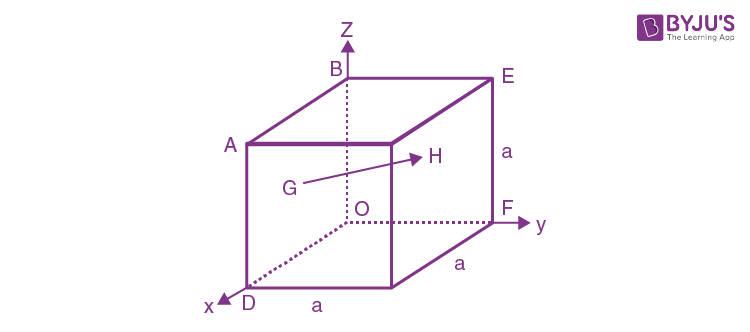1. a.
$$\frac{1}{2}a(\hat{k}-\hat{i})$$
2. b.
$$\frac{1}{2}a(\hat{i}-\hat{k})$$
3. c.
$$\frac{1}{2}a(\hat{j}-\hat{i})$$
4. d.
$$\frac{1}{2}a(\hat{j}-\hat{k})$$

Solution:

1. Position vector of G is

$$\vec{OG}= \frac{a}{2}\hat{i}+\frac{a}{2}\hat{k}$$

Position vector of H is

$$\vec{OH}= \frac{a}{2}\hat{j}+\frac{a}{2}\hat{k}$$

$$\vec{GH}= \frac{a}{2}(\hat{j}-\hat{i})$$

4. A piece of wood of mass 0.03 kg is dropped from the top of a building 100 m high. At the same time, a bullet of mass 0.02 kg is fired vertically upward with a velocity of 100 m/s from the ground the bullet gets embedded in the wooded piece after striking. Then the maximum height to which the combined system reaches above the building before falling below is: (take g = 10ms-2).

1. a. 40
2. b. 30
3. c. 20
4. d. 10

Solution:

1.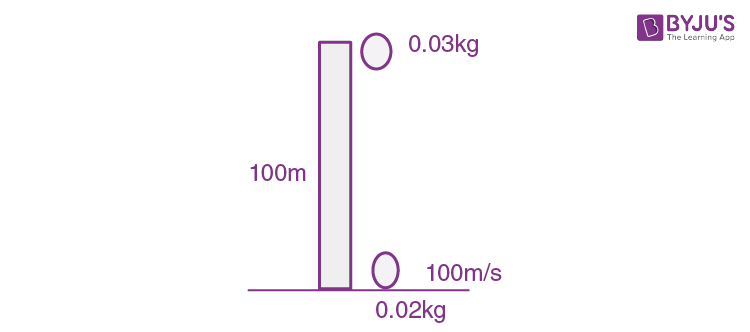Time taken by the particles to collide, t = d/Vrel = 100/100 = 1 sec

Before collision, speed of wood = gt = 10m/s

Before collision, speed of bullet = v-gt = 100-10 = 90 m/s

Conservation of linear momentum just before and after the collision = (0.02) (1v) + (0.02) (9v)

= (0.05)v

150 = 5v

v = 30 m/s

Max. height reached by body h = v2/2g

h = (30×30)/2×10 = 45 m

S = ut+(1/2)at2 = 0+(1/2)10 = 5m

So height above the tower = 45-5 = 40 m

5. A block of mass m is kept on a platform which starts from rest with constant acceleration g/2 upward, as shown in fig. Work done by normal reaction on block in time t is :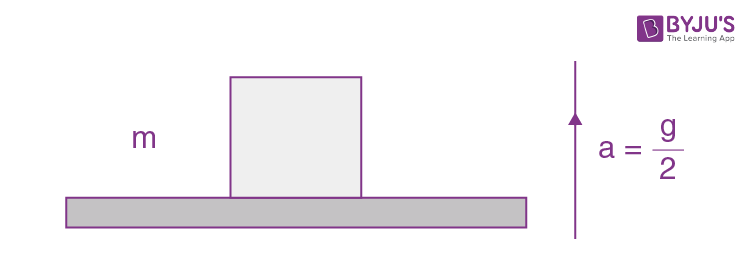1. a. 3mg2t2/8
2. b. -mg2t2/8
3. c. mg2t2/8
4. d. 0

Solution:

1. S = ut+(1/2)at2

ut = 0

a = g/2

S = (1/4)gt2

N-mg = mg/2

N = 3mg/2

Work done W = FS = NS

= (3mg/2)(gt2/4)

= 3mg2t2/8

6. A homogeneous solid cylindrical roller of radius R and mass M is pulled on a cricket pitch by a horizontal force. Assuming rolling without slipping, angular acceleration of the cylinder is:

1. a. 2F/3mR
2. b. 3F/2mR
3. c. F/3mR
4. d. F/2mR

Solution:

1. Considering pure rolling, acm = Rα

FR = (mR2 +(1/2) mR2 ) α

= (3/2)mR2α

α = 2F/3mR

7. To mop-clean a floor, a cleaning machine presses a circular mop of radius R vertically down with a total force F and rotates it with a constant angular speed about its axis. If the force F is distributed uniformly over the mop and the floor is μ, the torque, applied by the machine on the mop is :

1. a. μFR/6
2. b. μFR/3
3. c. μFR/2
4. d. 2μFR/3

Solution:

1. Force per unit length = F/πR2)2πxdx

Torque = F×r

=

$$\int_{0}^{R}\mu (\frac{F}{\pi R^{2}}2\pi x\; dx)x$$

=

$$\frac{2\mu F}{ R^{2}}\int_{0}^{R} x^{2}\; dx$$

=

$$\frac{2\mu F}{ R^{2}}\frac{R^{3}}{3}$$

=

$$\frac{2\mu FR}{ 3}$$

8. A satellite is moving with a constant speed v in circular orbit around the earth. An object of mass m is ejected from the satellite such that it just escapes from the gravitational pull of the earth. At the time of ejection, the kinetic energy of the object is

1. a. (1/2)mv2
2. b. mv2
3. c. (3/2)mv2
4. d. 2mv2

Solution:

1. Orbital velocity = v

Escape velocity = Ve

v = √(GM/R)

g = GM/R2

gR2 = GM

v = √gR

Ve = √(2gR)

= √2 v

Kinetic energy = (1/2)mVe2

= (1/2) m(√2v)2

= mv2

9. Water flows into a large tank with flat bottom at the rate of 10−4m3s−1. Water is also leaking out of a hole of area 1 cm2 at its bottom. If the height of the water in the tank remains steady, then this height is

1. a. 5.1 cm
2. b. 4 cm
3. c. 2.9 cm
4. d. 1.7 cm

Solution:

1. Water inflow rate = water outflow rate

Water inflow rate = 10-4

Water outflow rate = Au = 10-4×√(2gh)

10-4 = 10-4×√(2gh)

So h = 1/2g

= 1/(2×10)

= 1/20

= 5×10-2 m

= 5 cm

10. Three Carnot engines operate in series between a heat source at a temperature T1 and a heat sink at temperature T4 (see figure). There are two other reservoirs at temperature T2 and T3, as shown, with T1 >T2 >T3 >T4. The three engines are equally efficient if :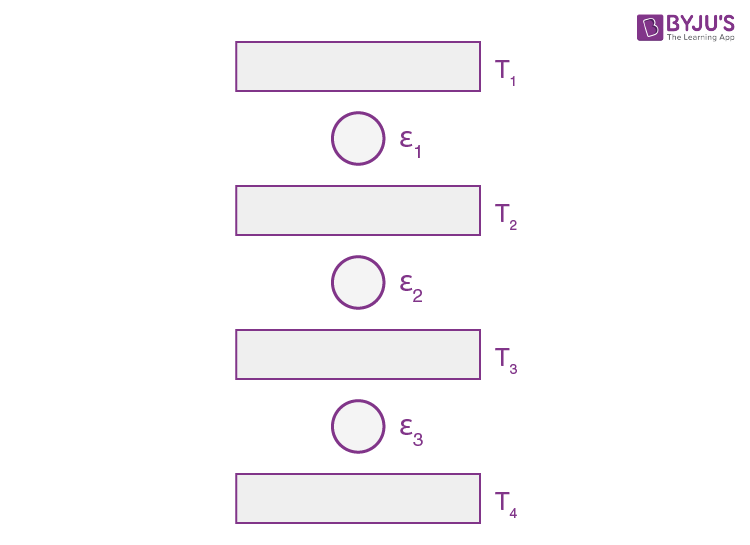1. a. T2 = (T1T42)1/3 ; T3 = (T12 T4)1/3
2. b. T2 = (T1T4)1/2 ; T3 = (T12 T4)1/3
3. c. T2 = (T12 T4)1/3 ; T3 = (T1 T42)1/3
4. d. T2 = (T13 T4)1/4 ; T3 = (T1 T43)1/4

Solution:

1. t1 = 1-(T2/T1)

= 1-(T3/T2)

= 1-(T4/T3)

So T2/T1 = T3/T2 = T4/T3

T2 = √(T1T3)

=

$$\sqrt{T_{1}\sqrt{T_{2}T_{4}}}$$

T3 =

$$\sqrt{T_{2}T_{4}}$$

T23/4 =

$$\sqrt{T_{1}^{\frac{1}{2}}}T_{4}^{\frac{1}{4}}$$

T2 = T12/3 T41/3

T2 = (T12 T4 )1/3

T3 = (T1 T42)1/3

11. A heat source at T = 103 K is connected to another heat reservoir at T = 102 K by a copper slab which is 1 m thick. Given that the thermal conductivity of copper is 0.1 WK-1m-1, the energy flux through it in steady state is :

1. a. 90 W/m2
2. b. 120 W/m2
3. c. 65 W/m2
4. d. 200 W/m2

Solution:

1. (dQ/dt) = kAΔt/l

(1/A)(dQ/dt) = (0.1×900)/1 = 90W/m2

12. A string of length 1 m and mass 5 g is fixed at both ends. The tension in the string is 8.0 N. The siring is set into vibration using an external vibrator of frequency 100 Hz. The separation between successive nodes on the string is close to __?

1. a. 10 cm
2. b. 16.6 cm
3. c. 20 cm
4. d. 33.3 cm

Solution:

1. The velocity of wave on string, V = √(T/μ)

= √(8×1000/5)

= 40 m/s

The wavelength of wave, λ = v/n = 40/100m

Separation between successive nodes, λ/2 = 20/100 m = 20 cm

13. A train moves towards a stationary observer with speed 34m/s. The train sounds a whistle and its frequency registered by the observer is f1. If the train's speed is reduced to 17m/s, the frequency registered is f2. If the speed of sound of 340m/s, then the ratio f1/f2 is

1. a. 18/7
2. b. 21/20
3. c. 20/19
4. d. 19/18

Solution:

1. f1 = f(v/v-vs)

= f(340/340-34)

= f(340/306)

f2 = f(340/340-17)

= f(340/323)

f1/f2 = 323/306 = 19/18

14. A parallel plate capacitor is of area 6 cm2 and a separation 3 mm. The gap is filled with three dielectric materials of equal thickness (see figure) with dielectric constant K1=10, K2 = 12 and K3 =14. The dielectric constant of a material which when fully inserted in above capacitor, gives same capacitance would be :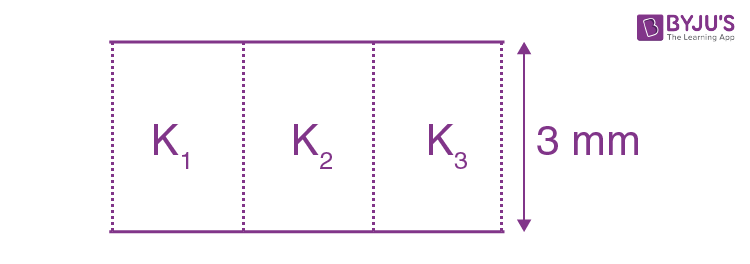1. a. 34
2. b. 12
3. c. 4
4. d. 14

Solution:

1. Let K be the dielectric constant of the material used.

So

$$\frac{10\varepsilon _{0}\frac{A}{3}}{d}+\frac{12\varepsilon _{0}\frac{A}{3}}{d}+\frac{14\varepsilon _{0}\frac{A}{3}}{d}= \frac{K\varepsilon _{0}A}{d}$$

So K = 36/3 = 12

15. Two electric dipoles, A,B with respective dipole moments

$$\vec{d_{A}}=-4qa\hat{i}$$
and
$$\vec{d_{B}}=-2qa\hat{i}$$
placed on the x-axis with a separation R, as shown in the figure.The distance from A at which both of them produce the same potential is:

1. a.
$$\frac{\sqrt{2}R}{\sqrt{2}-1}$$
2. b.
$$\frac{\sqrt{2}R}{\sqrt{2}+1}$$
3. c.
$$\frac{R}{\sqrt{2}+1}$$
4. d.
$$\frac{R}{\sqrt{2}-1}$$

Solution:

1. V = 4qa/(R+x)

= 2qa/x2

√2x = R+x

x = R/(√2-1)

distance =

$$\frac{R}{\sqrt{2}-1}+R = \frac{\sqrt{2}R}{\sqrt{2}-1}$$

16. A charge Q is distributed over three concentric spherical shells of radii a, b, c (a< b < c) such that their surface charge densities are equal to one another.

The total potential at a point at distance r from their common centre, where r < a, would be :

1. a.
$$\frac{Q}{4\pi \epsilon _{0}(a+b+c)}$$
2. b.
$$\frac{Q(a+b+c)}{4\pi \epsilon _{0}(a^{2}+b^{2}+c^{2})}$$
3. c.
$$\frac{Q(ab+bc+ca)}{12\pi \epsilon _{0}(abc)}$$
4. d.
$$\frac{Q(a^{2}+b^{2}+c^{2})}{4\pi \epsilon _{0}(a^{3}+b^{3}+c^{3})}$$

Solution:

1. Potential at point P, V =

$$\frac{kQ_{a}}{a}+\frac{kQ_{b}}{b}+\frac{kQ_{c}}{c}$$

Since Qa : Qb : Qc : :a 2 : b2: c2

Since σa = σb = σc

So Qa = (a2/a2+b2+c2) Q

Qb = (b2/a2+b2+c2) Q

Qc = (c2/a2+b2+c2) Q

V =

$$\frac{Q(a+b+c)}{4\pi \epsilon _{0}(a^{2}+b^{2}+c^{2})}$$

17. A uniform metallic wire of resistance 18 ohm is bent to form an equilateral triangle . The resistance across any two vertices of the triangle is (in ohm).

1. a. 8 ohm
2. b. 12 ohm
3. c. 4 ohm
4. d. 2 ohm

Solution:

1.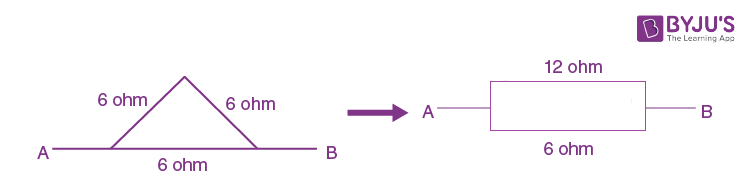Let Req be resistance between any two vertex.

1/Req = (1/12)+(1/6)

So Req = 4 ohm

18. In the given circuit the cells have zero internal resistance. The currents (in Amperes) passing through resistance R1, and R2 respectively, are: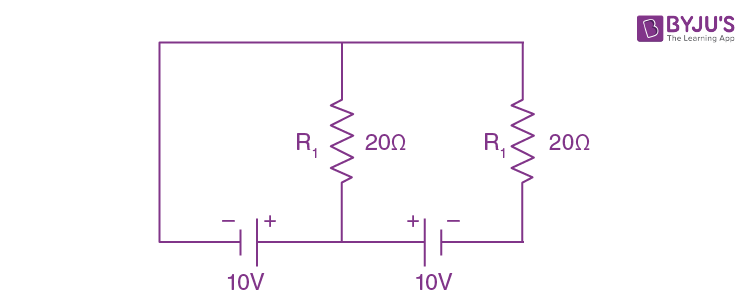1. a. 2,2
2. b. 0,1
3. c. 1,2
4. d. 0.5,0

Solution:

1. i1 = 10/20 = 0.5 A

i2 = 0

19. A solid metal cube of edge length 2 cm is moving in the positive y direction at a constant speed of 6 m/s. There is a uniform magnetic field of 0.1 T in the positive z direction. The potential difference between the two faces of the cube perpendicular to the x axis, is :

1. a. 1 mV
2. b. 6 mV
3. c. 12 mV
4. d. 2 mV

Solution:

1. The potential difference between two faces perpendicular to x axis will be

$$l.(\vec{V}\times \vec{B} )= 12 mV$$

20. An insulting thin rod of length l has a linear charge density

$$\rho (x)= \rho _{0}\frac{x}{l}$$
on it. The rod is rotated about an axis passing through the origin (x = 0) and perpendicular to the rod. If the rod makes n rotations per second, then the time averaged magnetic moment of the rod is :

1. a. (π/4)nρl3
2. b. nρl3
3. c. πnρl3
4. d. (π/3)nρl3

Solution:

1. Since M = NIA

dq = λdx and A = πx2

$$\int dm =\int n\frac{\rho _{0}(x)}{l}dx\pi x^{2}$$

$$M =n\rho _{0}\frac{\pi }{l}\int_{0}^{l}x^{3}dx= n\rho _{0}\frac{\pi }{l}\left [ \frac{l^{4}}{4} \right ]$$

$$M =\frac{n\rho _{0}\pi l^{3}}{4} \; or\; \frac{\pi }{4}n\rho l^{3}$$

21. A magnet of total magnetic moment

$$10^{-2}\hat{i}$$
A-m2 is placed in a time-varying magnetic field,
$$B\hat{i}(\cos \omega t)$$
where B = 1 Tesla and ω= 0.125 rad/s. The work done for reversing the direction of the magnetic moment at t = 1 second, is

1. a. 0.007 J
2. b. 0.02 J
3. c. 0.01 J
4. d. 0.028 J

Solution:

1. Work done, W =

$$(\Delta \vec{\mu }).\vec{B}$$

= 2×10-2×1 cos(0.125)

= 0.02 J

22. If the magnetic field of a plane electromagnetic wave is given by (The speed of light =3×108 m/sec)

$$B = 100\times 10^{-6}\sin \left ( 2\pi \times 2\times 10^{15} (t-\frac{x}{c})\right )$$
then the maximum electric field associated with it is

1. a. 4×104 N/C
2. b. 6×104 N/C
3. c. 3×104 N/C
4. d. 4.5×104 N/C

Solution:

1. E0 = B0×C

= 100×10-6×3×108

= 3×104N/C

23. A plano convex lens of refractive index μ1 and focal length f1 is kept in contact with another plano concave lens of refractive index μ2 and focal length f2. If the radius of curvature of their spherical faces is R each and f1 = 2f2, then μ1 and μ2 are related as :

1. a. μ1 + μ2 = 3
2. b. 2μ2- μ1 = 1
3. c. 2μ1- μ2 = 1
4. d. 3μ2- 2μ1 = 1

Solution:

1. Given f1 = 2f2

(1/2f2) = (1/f1) = (μ1-1)[(1/∞)-(1/-R)]

(1/f2) = (μ2-1)[(1/-R)-(1/∞)]

1-1)/R = (μ2-1)2R

⇒ 2μ12 = 1

24. In a Young's double slit experiment with slit separation 0.1 mm, one observes a bright fringe at angle 1/40 rad by using light of wavelength λ1. When the light of wavelength λ2 is used a bright fringe is seen at the same at the same angle in the same set up. Given that λ1 and λ2 are in visible range (380 nm to 740 nm), their values are :

1. a. 380 nm, 525 nm
2. b. 625 nm, 500 nm
3. c. 380 nm, 500 nm
4. d. 400 nm, 500 nm

Solution:

1. Path difference = d sinθ = dθ (approx)

= 0.1×(1/40)

= 2500 nm

For bright fringe, path difference must be integral multiple of λ.

So 2500 = nλ1 = mλ2

Hence λ1 = 625 (For n = 4)

λ2 = 500 (for m = 5)

25. In an electron microscope, the resolution that can be achieved is of the order of the wavelength of electrons used. To resolve a width of 7.5×10-12 m, the minimum electron energy required is close to :

1. a. 1 keV
2. b. 25 keV
3. c. 500 keV
4. d. 100 keV

Solution:

1. Given λ = 7.5×10-12

h = λ = h/P

P = h/λ

KE = P2/2m

= (h/λ)2/2m

(h/λ)2 =[(6.6×10-34)/ (7.5×10-12)]2

KE = [(6.6×10-34)/ (7.5×10-12)]2 /2×9.1×10-31

= 25 KeV

26. Using a nuclear counter the count rate of emitted particles from a radioactive source is measured. At t = 0 it was 1600 counts per second and t = 8 seconds it was 100 counts per second. The count rate observed, as counts per second, at t = 6 seconds is close to :

1. a. 150
2. b. 200
3. c. 360
4. d. 400

Solution:

1. At t = 0, A0 = dN/dt

= 1600 C/s

At t = 8s, A = 100 C/s

A/A0 = 1/16 in 8 seconds

Hence half life is t1/2 = 2 s

So the activity at t = 6 will be 1600/23

= 200 C/s

27. To get output ‘1’ at R, for the given logic gate circuit the input values must be :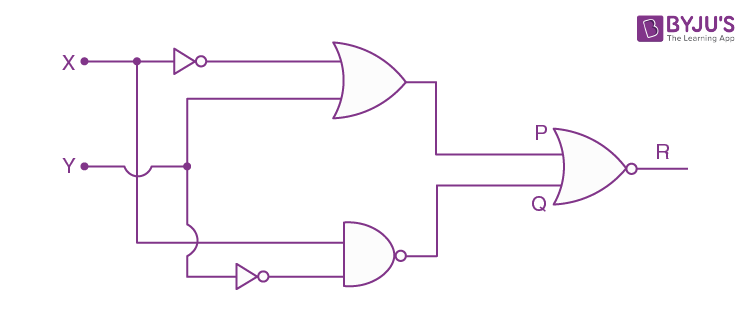1. a. X = 0, Y = 0
2. b. X = 0, Y = 1
3. c. X = 1, Y = 0
4. d. X = 1, Y = 1

Solution:

1. P =

$$\bar{x}+y$$

Q =

$$\overline{\bar{y}.x}$$
=
$$y+\bar{x}$$

O/P =

$$\overline{P+Q}$$

To make O/P

P+Q should be ‘O’

Hence X = 1 and Y = 0.

28. A TV transmission tower has a height of 140 m and the height of the receiving antenna is 40 m. What is the maximum distance upto which signals can be broad casted from this tower in LOS(Line of Sight) mode? (Given : radius of earth =6.4×106m)

1. a. 40 km
2. b. 80 km
3. c. 65 km
4. d. 48 km

Solution:

1. Given height of the transmission tower, ht = 140 m

Height of the receiving antenna, hr = 40 m

Radius of earth, R = 6.4×106 m

The max. distance to which the signal can be broadcasted is dmax = √(2Rht)+ √(2RhR)

Substituting the values, we get dmax = √(2×6.4×106 )×(√140+√40))

= 65000 m

= 65 km

29. A potentiometer wire AB having length L and resistance 12r is joined to a cell D of emf ε and internal resistance 3r is connected. The length AJ at which the galvanometer as shown in fig. shows no deflection is :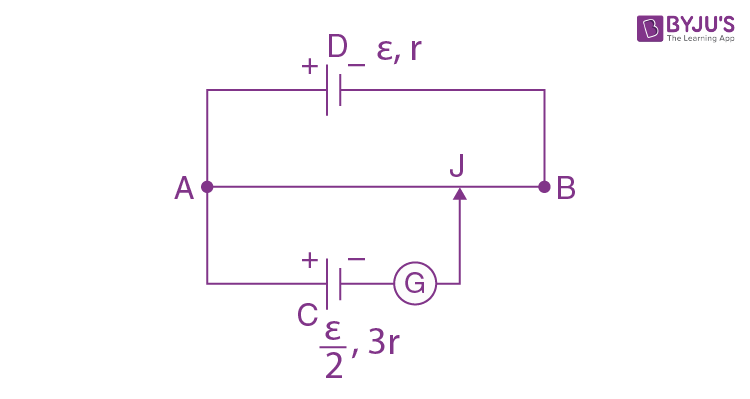1. a. (13/24) L
2. b. (5/12) L
3. c. (11/24) L
4. d. (11/12) L

Solution:

= (ε/13r)×(12r/L)

= 12ε/13L

(12ε/13L )x = ε/2

⇒ x = 13L/24

30. A 2 W carbon resistor is color coded with green, black, red and brown respectively. The maximum current which can be passed through this resistor is :

1. a. 0.4 mA
2. b. 20 mA
3. c. 63 mA
4. d. 100 mA

Solution:

1. P = i2R

For imax. R should be minimum.

From colour coding R = 50×102 ohm

imax= 20 mA

### JEE Main 2019 Physics Paper With Solutions January 10 Shift 1